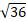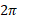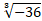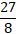# A WebQuest for grade 10 (Mathematics) Number System

Introduction

Lesson Aims:

• To expose learners to Real number System

Classify the following numbers according to types of numbers.

1.2.3.4.5. 67

( 5 marks)

Process
1. The teacher will give learners an indivisual activity.
2. Learners will use their workbooks and complete an activity.
3. Learners will use the given examples to an activity.
Evaluation

MEMO

1. Rational number
2. Irrational number
3. Irrational number
4. Rational number
5. Interger
Conclusion

This topic is the main foundation of Mathematics, understanding the number system and knowing types of numbers can help learners to easily understand Mathematics.

Credits

Grade 10 Learners Book, Classroom Mathematics (CAPS)

Teacher Page

MATHEBULA  DC

STUDENT NUMBER: 219062099